# Time Dependent Schrodinger Equation

The time dependent Schrodinger equation for one spatial dimension is of the formFor a free particle where U(x) =0 the wavefunction solution can be put in the form of a plane wave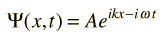For other problems, the potential U(x) serves to set boundary conditions on the spatial part of the wavefunction and it is helpful to separate the equation into the time-independent Schrodinger equation and the relationship for time evolution of the wavefunction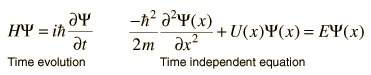Index

Schrodinger equation concepts

 HyperPhysics***** Quantum Physics R Nave
Go Back

# Free-Particle Wave Function

For a free particle the time-dependent Schrodinger equation takes the form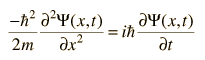and given the dependence upon both position and time, we try a wavefunction of the form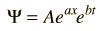Presuming that the wavefunction represents a state of definite energy E, the equation can be separated by the requirementProceeding separately for the position and time equations and taking the indicated derivatives: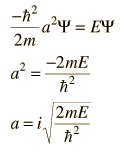Treating the system as a particle where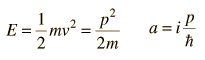Now using the De Broglie relationship and the wave relationship: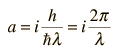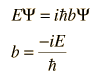Treating the system as a wave packet, or photon-like entity where the Planck hypothesis gives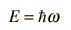we can evaluate the constant bThis gives a plane wave solution: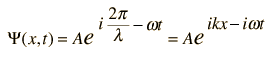More detail
Free particle approach to the Schrodinger equation
Index

Schrodinger equation concepts

 HyperPhysics***** Quantum Physics R Nave
Go Back

# Free Particle Waves

The general free-particle wavefunction is of the formwhich as a complex function can be expanded in the formEuler relationship.

Either the real or imaginary part of this function could be appropriate for a given application. In general, one is interested in particles which are free within some kind of boundary, but have boundary conditions set by some kind of potential. The particle in a box problem is the simplest example.

The free particle wavefunction is associated with a precisely known momentum: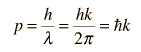but the requirement for normalization makes the wave amplitude approach zero as the wave extends to infinity (uncertainty principle).

 Tunneling Barrier penetration
Free particle approach to the Schrodinger equation
Index

Schrodinger equation concepts

 HyperPhysics***** Quantum Physics R Nave
Go Back

# Time Independent Schrodinger Equation

The time independent Schrodinger equation for one dimension is of the form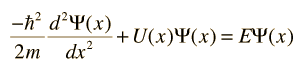where U(x) is the potential energy and E represents the system energy. It has a number of important physical applications in quantum mechanics. A key part of the application to physical problems is the fitting of the equation to the physical boundary conditions.

The time independent equation is readily generalized to three dimensions, and is often used in spherical polar coordinates.

Index

Schrodinger equation concepts

 HyperPhysics***** Quantum Physics R Nave
Go Back

# Energy Eigenvalues

To obtain specific values for energy, you operate on the wavefunction with the quantum mechanical operator associated with energy, which is called the Hamiltonian. The operation of the Hamiltonian on the wavefunction is the Schrodinger equation. Solutions exist for the time-independent Schrodinger equation only for certain values of energy, and these values are called "eigenvalues" of energy.For example, the energy eigenvalues of the quantum harmonic oscillator are given by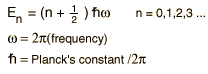The lower vibrational states of diatomic molecules often fit the quantum harmonic oscillator model with sufficient accuracy to permit the determination of bond force constants for the molecules.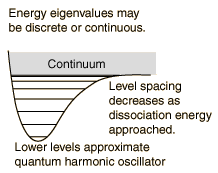While the energy eigenvalues may be discrete for small values of energy, they usually become continuous at high enough energies because the system can no longer exist as a bound state. For a more realistic harmonic oscillator potential (perhaps representing a diatomic molecule), the energy eigenvalues get closer and closer together as it approaches the dissociation energy. The energy levels after dissociation can take the continuous values associated with free particles.
Index

Schrodinger equation concepts

 HyperPhysics***** Quantum Physics R Nave
Go Back

# 1-D Schrodinger Equation

The time-independent Schrodinger equation is useful for finding energy values for a one dimensional systemConceptual comments

This equation is useful for the particle in a box problem which yields: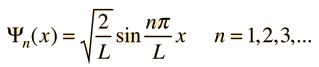To evaluate barrier penetration, the wavefunction inside a barrier is calculated to be of form:The quantum harmonic oscillator in one dimension yields: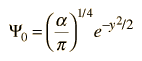This is the ground state wavefunction, where y is the displacement from equilibrium.

### 3-dimensional Shrodinger equation

Index

Schrodinger equation concepts

 HyperPhysics***** Quantum Physics R Nave
Go Back

# Physical Boundary Conditions and the Uniqueness Theorem

For physical applications of quantum mechanics that involve the solution of the Schrodinger equation, such as those of the time independent Schrodinger equation, one must find specific mathematical solutions that fit the physical boundary conditions of the problem.

One very important idea in differential equations is the "uniqueness theorem", which basically says that if you can find a solution to the differential equation that fits the physical boundary conditions of the problem you are considering, then that is in fact the correct solution. That leads to typical comments from students of differential equations like "if you can guess the solution and force it to fit the boundary conditions, then you have the right answer."

The time-independent Schrodinger equation is used for a number of practical problems. Systems with bound states are related to the quantum mechanical "particle in a box", barrier penetration is important in radioactive decay, and the quantum mechanical oscillator is applicable to molecular vibrational modes.

If, for example, you go to the "particle in a box", you find that for an infinite-walled box the wavefunction must equal zero at the edges of the box, and you can propose a sine wave solution and force it to fit the boundary conditions. How would you know to propose a sine wave solution? Well, in many areas of mathematics and science we "stand on the shoulders of giants" and can make use of the fact that many in the past have explored these kinds of problems and can suggest to us the types of solutions to try. This is part of the nature of applying differential equations to physical problems.

Index

Schrodinger equation concepts

 HyperPhysics***** Quantum Physics R Nave
Go Back